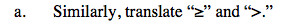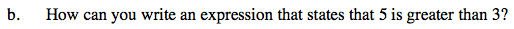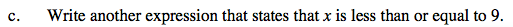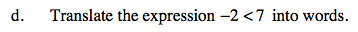### Home > CAAC > Chapter 8 > Lesson 8.2.3 > Problem8-70

8-70.
1. The "≤" symbol represents “less than or equal to,” while the “<” symbol represents “less than.” Homework Help ✎

1. Similarly, translate “>” and “>.”

2. How can you write an expression that states that 5 is greater than 3?

3. Write another expression that states that x is less than or equal to 9.

4. Translate the expression −2 < 7 into words.The symbol ≥ represents greater than or equal to, while the > symbol represents greater than.5 > 3Follow the steps in part (b).−2 is less than 7